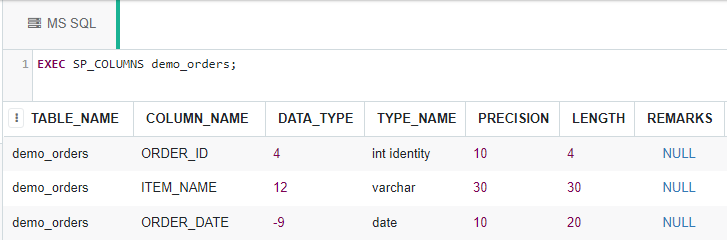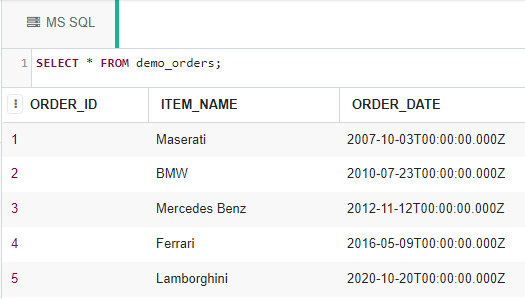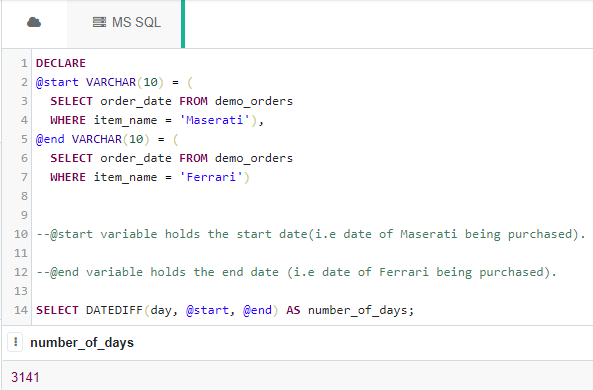# SQL Query to Calculate Total Number of Days Between Two Specific Dates

• Last Updated : 23 Apr, 2021

Here we will see, how to calculate the number of days between the two given dates with the help of SQL query using DATEDIFF() function.

For the purpose of demonstration, we will be creating a demo_orders table in a database called “geeks“.

### Creating the Database:

Use the below SQL statement to create a database called geeks:

`CREATE DATABASE geeks;`

### Using the Database:

Use the below SQL statement to switch the database context to geeks:

`USE geeks;`

### Table Definition:

We have the following demo_table in our geeks database.

```CREATE TABLE demo_orders(
ORDER_ID INT IDENTITY(1,1) PRIMARY KEY,
ITEM_NAME VARCHAR(30) NOT NULL,
ORDER_DATE DATE
);```

You can use the below statement to query the description of the created table:

`EXEC SP_COLUMNS demo_orders;`### Adding data to the table:

Use the below statement to add data to the demo_orders table:

```INSERT INTO demo_orders --no need to mention columns explicitly as we are inserting into
--all columns and ID gets
--automatically incremented.
VALUES
('Maserati', '2007-10-03'),
('BMW', '2010-07-23'),
('Mercedes Benz', '2012-11-12'),
('Ferrari', '2016-05-09'),
('Lamborghini', '2020-10-20');```

To verify the contents of the table use the below statement:

`SELECT * FROM demo_orders;`Now let’s find the number of months between the dates of the order of ‘Maserati’ and ‘Ferrari’ in the table using DATEDIFF() function.

Below is the syntax for the DATEDIFF() function to find the no. of days between two given dates.

`Syntax: DATEDIFF(day or dy or y, <start_date>, <end_date>);`

Example:

```DECLARE
@start VARCHAR(10) = (
SELECT order_date FROM demo_orders
WHERE item_name = 'Maserati'),
@end VARCHAR(10) = (
SELECT order_date FROM demo_orders
WHERE item_name = 'Ferrari')

--@start variable holds the start date(i.e date of Maserati being purchased).

--@end variable holds the end date (i.e date of Ferrari being purchased).

SELECT DATEDIFF(day, @start, @end) AS number_of_days;```

Output:My Personal Notes arrow_drop_up